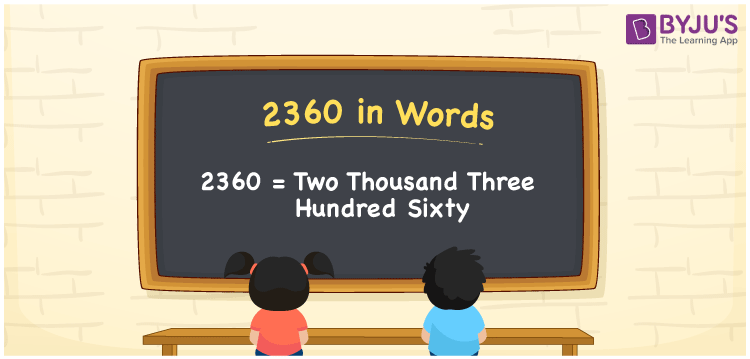# 2360 in words

2360 in words is written as Two Thousand Three Hundred and Sixty. 2360 represents the count or value. The article on Counting Numbers can give you an idea about count or counting. The number 2360 is used in expressions that relate to money, distance, length and others. Let us consider an example for 2360. ”The distance between Mysuru and Delhi is almost Two Thousand Three Hundred and Sixty kilometers.”

 2360 in words Two Thousand Three Hundred and Sixty Two Thousand Three Hundred and Sixty in Numbers 2360

## 2360 in English Words## How to Write 2360 in Words?

We can convert 2360 to words using a place value chart. The number 2360 has 4 digits, so let’s make a chart that shows the place value up to 4 digits.

 Thousands Hundreds Tens Ones 2 3 6 0

Thus, we can write the expanded form as:

2 × Thousand + 3 × Hundred + 6 × Ten + 0 × One

= 2 × 1000 + 3 × 100 + 6 × 10 + 0 × 1

= 2360

= Two Thousand Three Hundred and Sixty.

2360 is the natural number that is succeeded by 2359 and preceded by 2361.

2360 in words – Two Thousand Three Hundred and Sixty.

Is 2360 an odd number? – No.

Is 2360 an even number? – Yes.

Is 2360 a perfect square number? – No.

Is 2360 a perfect cube number? – No.

Is 2360 a prime number? – No.

Is 2360 a composite number? – Yes.

## Solved Example

1. Write the number 2360 in expanded form

Solution: 2 × 1000 + 3 × 100 + 6 × 10 + 0 × 1

We can write 2360 = 2000 + 300 + 60 + 0

= 2 × 1000 + 3 × 100 + 6 × 10 + 0 × 1

## Frequently Asked Questions on 2360 in words

Q1

### How to write 2360 in words?

2360 in words is written as Two Thousand Three Hundred and Sixty.
Q2

### Is 2360 a perfect square number?

No. 2360 is not a perfect square number.
Q3

### Is 2360 a prime number?

No. 2360 is not a prime number.